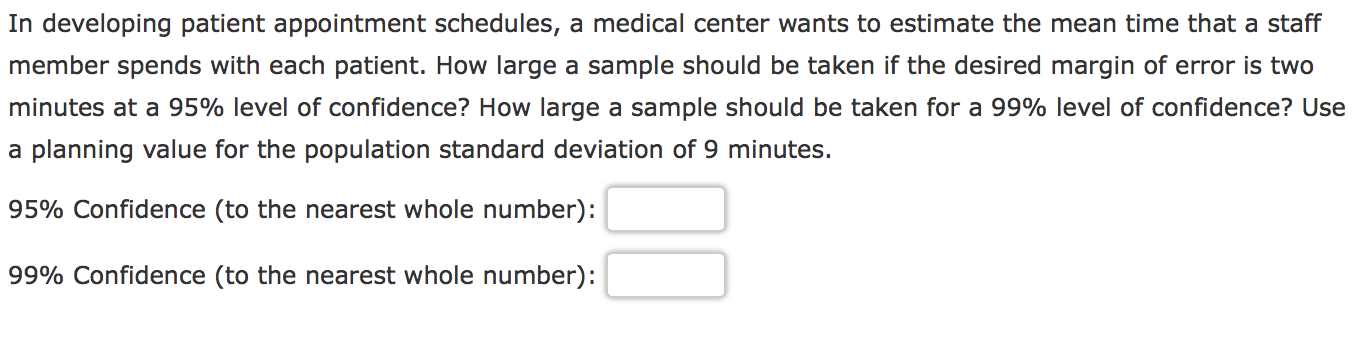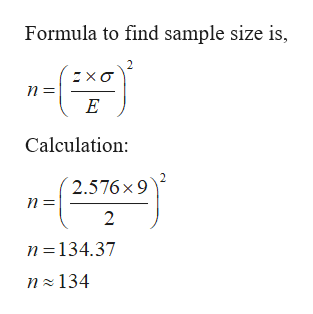# In developing patient appointment schedules, a medical center wants to estimate the mean time that a staffmember spends with each patient. How large a sample should be taken if the desired margin of error is twominutes at a 95% level of confidence? How large a sample should be taken for a 99% level of confidence? Usea planning value for the population standard deviation of 9 minutes.95% Confidence (to the nearest whole number):99% Confidence (to the nearest whole number)

Question
21 viewshelp_outlineImage TranscriptioncloseIn developing patient appointment schedules, a medical center wants to estimate the mean time that a staff member spends with each patient. How large a sample should be taken if the desired margin of error is two minutes at a 95% level of confidence? How large a sample should be taken for a 99% level of confidence? Use a planning value for the population standard deviation of 9 minutes. 95% Confidence (to the nearest whole number): 99% Confidence (to the nearest whole number) fullscreen
check_circle

Step 1

Given:

Standard deviation = σ = 9

Margin of error = 2

To find sample size for given confidence level.

Step 2

For 95%,

First need to find z for 95%.

P(Z≤z) = 0.95

Using standard normal table,

Z = 1.96

Formula to find sample size is,

Step 3

For 99%,

First need to find z for 995%.

P(Z≤z) = 0.99

Usi...help_outlineImage TranscriptioncloseFormula to find sample size is, хо п - n E Calculation: 2.576 x 9 п- n 134.37 n 134 fullscreen

### Want to see the full answer?

See Solution

#### Want to see this answer and more?

Solutions are written by subject experts who are available 24/7. Questions are typically answered within 1 hour.*

See Solution
*Response times may vary by subject and question.
Tagged in

### Hypothesis Testing